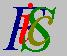Mauro Murzi's pages on Philosophy of Science - Quantum mechanics
Simplifying first Schrödinger equationFirst Schrödinger equation Bohr energy levels# [3. Schrödinger equations.]

## Simplifying first Schrödinger equation.

With few mathematical passages and using the constant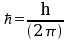the first Schrödinger equation for the energy E becomes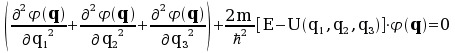.

The equation can be simplified with the introduction of the Laplace operator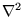as an abbreviation for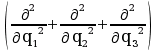(the symbol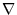is called the nabla operator). The first Schrödinger equation for the energy E can be written in the compact form: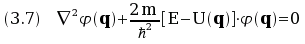.First Schrödinger equation Bohr energy levels